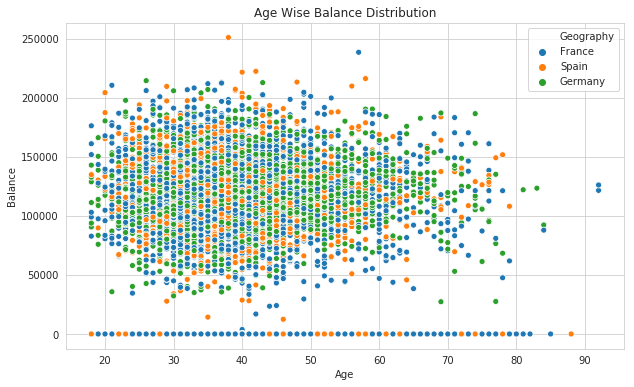# Mastering Data Exploration: An In-Depth Guide with Code Examples in Python### Introduction: The Significance of Data Exploration in Data Science

Data exploration is a critical first step in any data analysis project, as it allows practitioners to gain insights into the structure, quality, and relationships within a dataset. This process often includes examining summary statistics, visualizing data, identifying outliers and missing values, and performing feature engineering to prepare the data for modeling. In this comprehensive article, we will outline an 11-step guide to data exploration, complete with code examples, to help you effectively analyze and understand your data.

```import pandas as pd
import numpy as np

### 2. Understanding the Dataset Structure

Examine the structure of the dataset by displaying the first few rows and checking the dimensions, column names, and data types.

```data.head()
data.shape
data.columns
data.dtypes```

### 3. Summary Statistics

Calculate summary statistics for the numerical and categorical variables using the describe() and value_counts() functions.

```
data.describe()
data[“categorical_variable”].value_counts()

```

### 4. Identifying Missing Values

Identify missing values in the dataset using the isnull() function, and decide whether to impute or remove these values based on the nature and extent of the missing data.

```
data.isnull().sum()

```

### 5. Univariate Analysis

Perform univariate analysis to examine the distribution of each variable using histograms, box plots, or bar charts.

```import matplotlib.pyplot as plt
import seaborn as sns```
```sns.histplot(data[“numerical_variable”])
sns.boxplot(data[“numerical_variable”])
sns.countplot(data[“categorical_variable”])```

### 6. Bivariate Analysis

Conduct bivariate analysis to explore the relationships between pairs of variables using scatter plots, correlation matrices, or cross-tabulations.

```
sns.scatterplot(x=”numerical_variable1", y=”numerical_variable2", data=data)
sns.heatmap(data.corr(), annot=True)
pd.crosstab(data[“categorical_variable1”], data[“categorical_variable2”])

```

### 7. Identifying and Treating Outliers

Detect outliers in the dataset using box plots, IQR, or Z-score methods, and decide whether to remove or transform these values based on their impact on the analysis.

```
Q1 = data[“numerical_variable”].quantile(0.25)
Q3 = data[“numerical_variable”].quantile(0.75)

IQR = Q3 — Q1

outliers = data[(data[“numerical_variable”] < (Q1–1.5 * IQR)) | (data[“numerical_variable”] > (Q3 + 1.5 * IQR))]

```

### 8. Feature Engineering

Create new features or transform existing features to improve their relevance or interpretability in the analysis. This may include creating dummy variables, binning, or applying mathematical transformations.

```
data[“new_feature”] = data[“numerical_variable”] ** 2
data = pd.get_dummies(data, columns=[“categorical_variable”])

```

### 9. Feature Scaling

Normalize or standardize numerical features to ensure they are on the same scale, particularly if they will be used as inputs to a machine learning model.

`from sklearn.preprocessing import StandardScaler`
```scaler = StandardScaler()
data[“scaled_numerical_variable”] = scaler.fit_transform(data[[“numerical_variable”]])```

### 10. Dimensionality Reduction

Reduce the dimensionality of the dataset using techniques such as Principal Component Analysis (PCA) or t-distributed Stochastic Neighbor Embedding (t-SNE) to simplify the analysis and improve the performance of machine learning models.

`from sklearn.decomposition import PCA`
```pca = PCA(n_components=2)
data_pca = pca.fit_transform(data[[“numerical_variable1”, “numerical_variable2”]])```

### 11. Saving the Preprocessed Dataset

After completing the data exploration and preprocessing steps, save the cleaned and transformed dataset to a new file for further analysis or modeling.

```
data.to_csv(“cleaned_data.csv”, index=False)

```

### Conclusion: The Importance of Thorough Data Exploration in Data Science

Data exploration is a vital step in the data science process, as it allows practitioners to understand the quality, structure, and relationships within a dataset. By following this 11-step guide and employing the provided code examples, you can effectively explore and preprocess your data, laying a strong foundation for subsequent analysis and modeling. By mastering data exploration, you can ensure more accurate, reliable, and interpretable results in your data science projects, driving better decision-making and insights across various domains.

# Personal Career & Learning Guide for Data Analyst, Data Engineer and Data Scientist

## Applied Machine Learning & Data Science Projects and Coding Recipes for Beginners

A list of FREE programming examples together with eTutorials & eBooks @ SETScholars

# Projects and Coding Recipes, eTutorials and eBooks: The best All-in-One resources for Data Analyst, Data Scientist, Machine Learning Engineer and Software Developer

Topics included:Classification, Clustering, Regression, Forecasting, Algorithms, Data Structures, Data Analytics & Data Science, Deep Learning, Machine Learning, Programming Languages and Software Tools & Packages.
(Discount is valid for limited time only)## Find more … …

Unveiling the Power of Data: A Comprehensive Guide to Data Exploration with R Programming Language

Data Cleaning in R – remove outliers in R

Transforming Industries with AI: A Comprehensive Exploration of Artificial Intelligence Applications Across Sectors